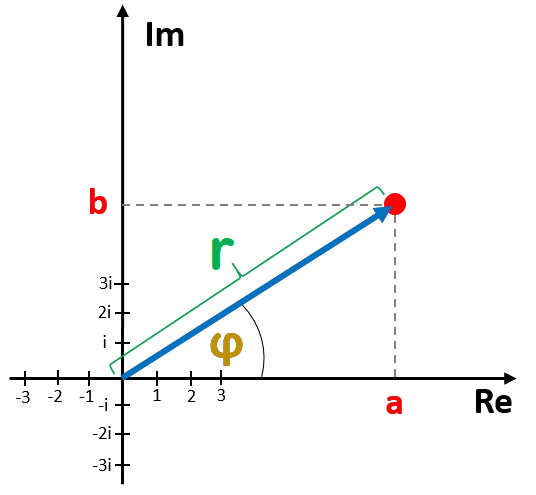# Polar Form Calculator

Created by Anna Szczepanek, PhD
Reviewed by Komal Rafay
Last updated: May 04, 2023

Omni's polar form calculator can help whenever you want to convert a complex number from rectangular to polar form. Scroll down to find a short article explaining what the different forms of a complex number are all about and what the polar form conversion formulas look like. Believe it or not, converting to polar form can be fun!

## What are the forms of a complex number?

The two main forms of a complex number are polar and rectangular. Usually, you first encounter the rectangular form z = a + bi, where a and b are the real part and imaginary part of z, respectively. They are both real numbers!

The polar form describes z by r × exp(φi), where:

• The magnitude r is the distance from the origin (0,0) to z; and
• The argument φ is the angle between the real axis (x-axis) and the radius connecting the origin (0,0) and z.

Look at the picture to better understand what the polar form is:## How do I convert from rectangular to polar form?

To convert a complex number from the rectangular a + bi to polar form z, we use the formulas:

r = √(a² + b²)

and

φ = atan2(b, a).

If a > 0, then atan2(b, a) = arctan(b / a). However, if a < 0, then atan(b /a) does not give us the correct angle - it must be corrected by ±π to send us into the correct quadrant of the plane. Formally atan2(b, a) is defined as:

• atan(b / a) if a > 0;
• atan(b / a) + π if a < 0 ≤ b;
• atan(b / a) - π if a,b < 0;
• π/2 if a = 0 < b;
• -π/2 if b < 0 = a; and
• remains undefined if x = y = 0.

These are exactly the formulas behind Omni's polar form converter.

## How to use this polar form calculator?

To use this polar form converter, take a complex number in the rectangular form a + bi and input a and b into the respective fields of our tool. The two ingredients of the polar form will appear immediately in their fields: the magnitude r and phase (argument) φ. Take them and write down the polar form r × exp(iφ) of your number.

This can't get any easier!

## Omni tools for complex numbers

Complex numbers can find you in sooo many areas of science! Omni is well aware of that - we have built a whole collection of tools addressing different problems related to complex numbers. Make sure to check out at least a few of the following tools:

## FAQ

### How do I convert from trigonometric to polar form?

To convert a complex number from trigonometric to polar form, you need to:

1. Make sure your number is written as z = r × cos(φ) + r × i × sin(φ)].
2. Extract the magnitude r and the argument φ.
3. Write down your number as r × exp(φi).
4. If in doubt, use an online polar form calculator.

### What is the polar form of -1?

The answer is exp(πi). To derive this answer, observe that the modulus (magnitude) of -1 is equal to 1. Next, we must determine φ such that cos(φ) = -1 and sin(φ) = 0. We easily verify that φ = π satisfies these conditions. Hence, the whole number reads 1 × exp(πi), as claimed.

Anna Szczepanek, PhD
Rectangular form a + bi
Real part (a)
Imaginary part (b)
Polar form r × exp(iφ)
Magnitude (r)
Phase (φ)
deg
People also viewed…

Addiction calculator tells you how much shorter your life would be if you were addicted to alcohol, cigarettes, cocaine, methamphetamine, methadone, or heroin.

### Grams to cups

The grams to cups calculator converts between cups and grams. You can choose between 20 different popular kitchen ingredients or directly type in the product density.